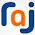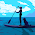VHDL coding tips and tricks: Measure the time period/Frequency of an input Pulse

## Monday, September 13, 2010

### Measure the time period/Frequency of an input Pulse

This article is about how to find the time period of an input pulse using a simple counter and some adders.In some applications you may have a pulse input which has unknown or varying frequency.And you may need to find out this frequency.In this design I have two parts.
First part is a counter which keeps on incrementing at the system clock frequency.When this counter reaches its maximum value it resets automatically and counts up again.The second part is triggered at every positive edge of the pulse input.So this part is triggered once every clock cycle of the pulse.In the second part we simply subtracts the last count from the current count to get the time period of the pulse in terms of the time period of the system clock.
For example if you see the simulation waveform I have attached at the bottom of this page you can see how this works:
1) pulse goes to '1' at 10 ns. Prev_Count is made "1000" which is the curr_count at 10 ns.
2) pulse goes to '0' at 110 ns. Nothing happens here. But all this time curr_count keeps on counting with the system clock.
3) pulse goes to '1' at 160 ns. Now we calculate (Curr_count - Prev_count ) = (16000 - 1000) =15000. This is how we get 15000 as the time period of the pulse input in terms of system clock. For getting the exact time period is ns you have to multiply the time period of system clock with the calculated value. Here we multiply 15000 with 10 ps = 150 ns.
4)For all this to work with good precision the frequency of the system clock should be very high compared to the frequency of pulse input. Otherwise the module will output the time period as "0" and a '1' will be asserted in the ERR_O output.
5)Whenever we calculate the time period of the pulse we update the prev_count with the current value of count.
6)Note that at time 1460.004 ns, at the new positive edge of pulse cycle we have a new time period calculated which is 130000 in relative terms or 1300 ns in absolute scale.
The code for the design is given below:

library ieee;
use ieee.std_logic_1164.all;
use ieee.numeric_std.all;
entity pulse_counter is
port ( DAT_O : out unsigned(47 downto 0);
ERR_O : out std_logic;  --This is '1' if the pulse freq is more than clk freq.
Pulse_I : in std_logic;
CLK_I : in std_logic
);
end pulse_counter;
architecture Behavioral of pulse_counter is
signal Curr_Count,Prev_Count : unsigned(47 downto 0):=(others => '0');
begin
--Increment Curr_Count every clock cycle.This is the max freq which can be measured by the module.
process(CLK_I)
begin
if( rising_edge(CLK_I) ) then
Curr_Count <= Curr_Count + 1;
end if;
end process;
--Calculate the time period of the pulse input using the current and previous counts.
process(Pulse_I)
begin
if( rising_edge(Pulse_I) ) then
--These different conditions eliminate the count overflow problem
--which can happen once the module is run for a long time.
if( Prev_Count < Curr_Count ) then
DAT_O <= Curr_Count - Prev_Count;
ERR_O <= '0';
elsif( Prev_Count > Curr_Count ) then
--X"F_F" is same as "1111_1111".
--'_' is added for readability.
DAT_O <= X"1_0000_0000_0000" - Prev_Count + Curr_Count;
ERR_O <= '0';
else
DAT_O <= (others => '0');
ERR_O <= '1';  --Error bit is inserted here.
end if;
Prev_Count <= Curr_Count;  --Re-setting the Prev_Count.
end if;
end process;
end Behavioral;

The testbench code used for testing the design is given below:

LIBRARY ieee;
USE ieee.std_logic_1164.ALL;
USE ieee.numeric_std.ALL;
ENTITY tb IS
END tb;
ARCHITECTURE behavior OF tb IS
--Inputs
signal Pulse_I : std_logic := '0';
signal CLK_I : std_logic := '0';
--Outputs
signal DAT_O : unsigned(47 downto 0);
signal ERR_O : std_logic;
-- Clock period definitions
constant CLK_I_period : time := 10 ps;
BEGIN
-- Instantiate the Unit Under Test (UUT)
uut: entity work.pulse_counter PORT MAP (
DAT_O => DAT_O,
ERR_O => ERR_O,
Pulse_I => Pulse_I,
CLK_I => CLK_I
);
-- Clock process definitions
CLK_I_process :process
begin
CLK_I <= '0';
wait for CLK_I_period/2;
CLK_I <= '1';
wait for CLK_I_period/2;
end process;
-- Stimulus process
stim_proc: process
begin
wait for 10 ns;
--1  (time period is 15000*10 ps here)
Pulse_I <= '1';
wait for 100 ns;
Pulse_I <= '0';
wait for 50 ns;
Pulse_I <= '1';
--2  (Error because freq of pulse is less than system clock)
wait for 3 ps;
Pulse_I <= '0';
wait for 1 ps;
Pulse_I <= '1';
--3  (time period is 130000*10 ps here)
wait for 300 ns;
Pulse_I <= '0';
wait for 1000 ns;
Pulse_I <= '1';
wait;
end process;
END;

The simulation wave form is shown below:
Markers are added in the waveform for clearly understanding the positive edges of the pulse wave.Go through the wave form along with the codes and the explanation given above.

#### 5 comments:

1.really helpful!

2.hello my friend, i dont understand this line:
DAT_O <= X"1_0000_0000_0000" - Prev_Count + Curr_Count;
and I dont understand why you use unsigned numbers. Can you help me? thank you

1.then only + operator support,otherwise error will come.so unsigned operation is used here.

3.X"1_0000_0000_0000" this portion is giving error... using this"1000000000000" no error but output is not as expected only error_o is 1 rest all are zero in tb

4.Thanks for you example. Can i ask why the X"1000" isnt X"0000"?

Cheers